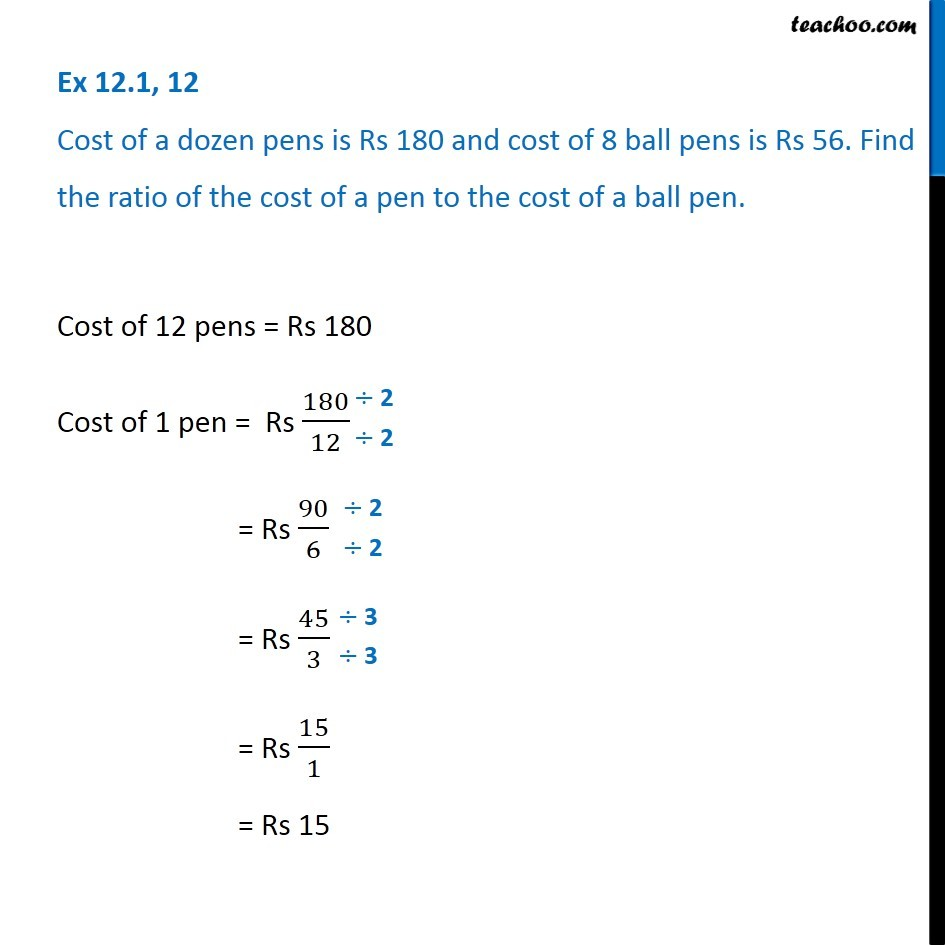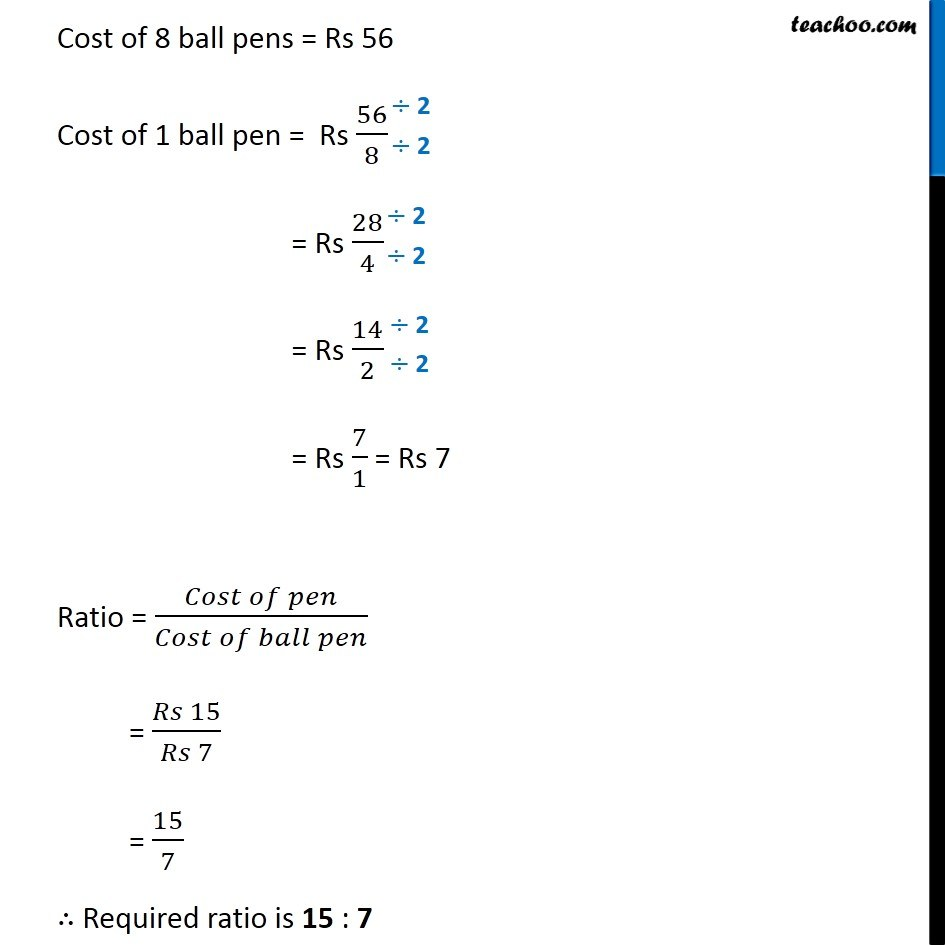Ratios

Chapter 12 Class 6 Ratio and Proportion
Concept wiseLearn in your speed, with individual attention - Teachoo Maths 1-on-1 Class

### Transcript

Ex 12.1, 12 Cost of a dozen pens is Rs 180 and cost of 8 ball pens is Rs 56. Find the ratio of the cost of a pen to the cost of a ball pen. Cost of 12 pens = Rs 180 Cost of 1 pen = Rs 180/12 = Rs 90/6 = Rs 45/3 = Rs 15/1 = Rs 15 Cost of 8 ball pens = Rs 56 Cost of 1 ball pen = Rs 56/8 = Rs 28/4 = Rs 14/2 = Rs 7/1 = Rs 7 Ratio = (𝐶𝑜𝑠𝑡 𝑜𝑓 𝑝𝑒𝑛)/(𝐶𝑜𝑠𝑡 𝑜𝑓 𝑏𝑎𝑙𝑙 𝑝𝑒𝑛) = (𝑅𝑠 15)/(𝑅𝑠 7) = 15/7 ∴ Required ratio is 15 : 7